﻿ 關註潛在的整數越界問題_ZenDei技術網路在線

# 關註潛在的整數越界問題# 比較器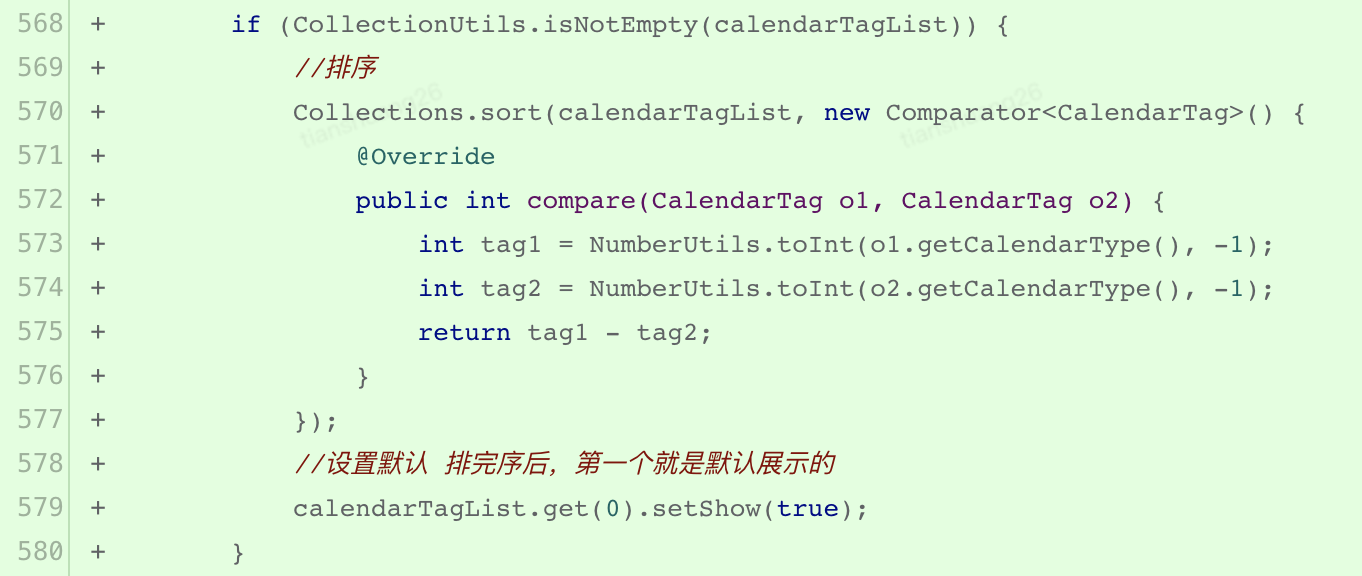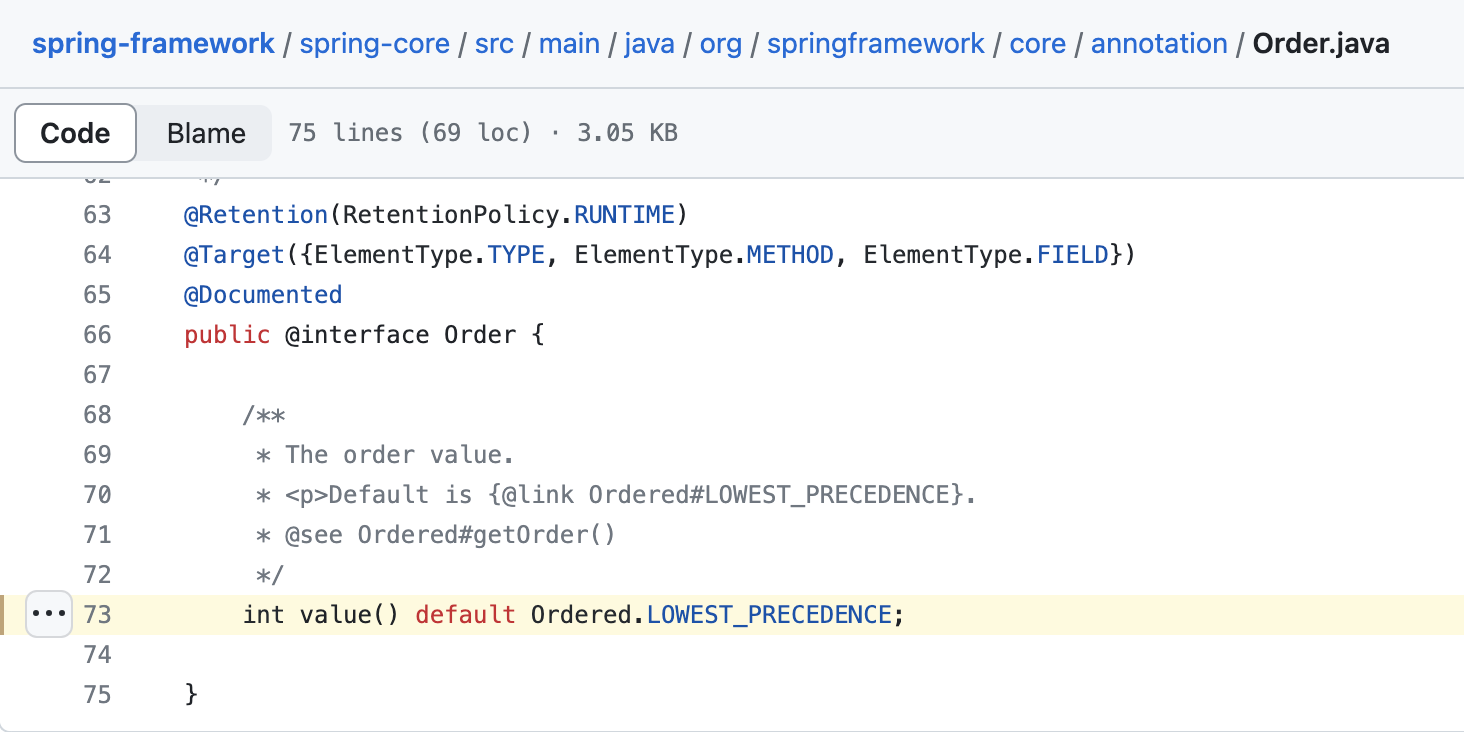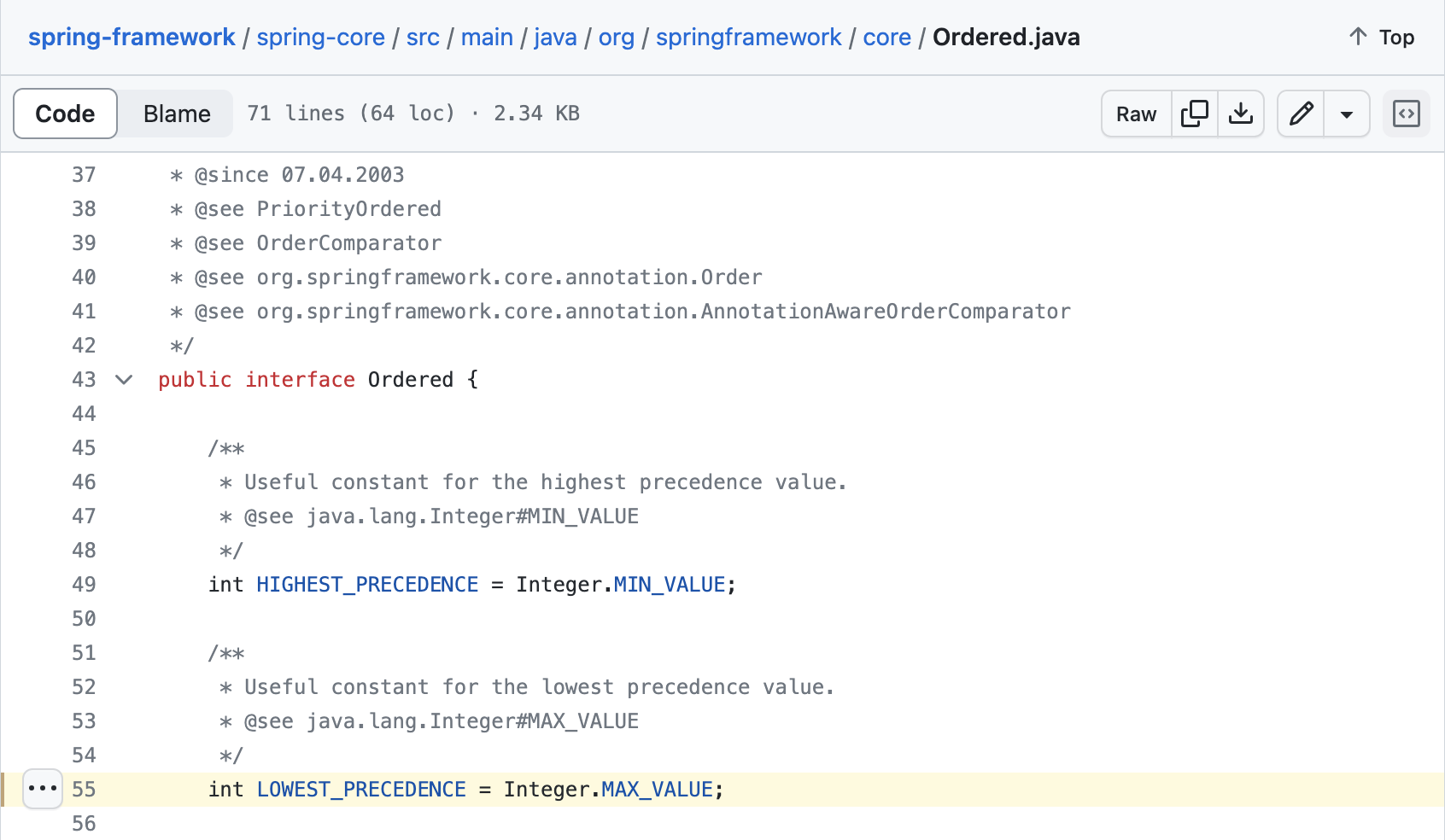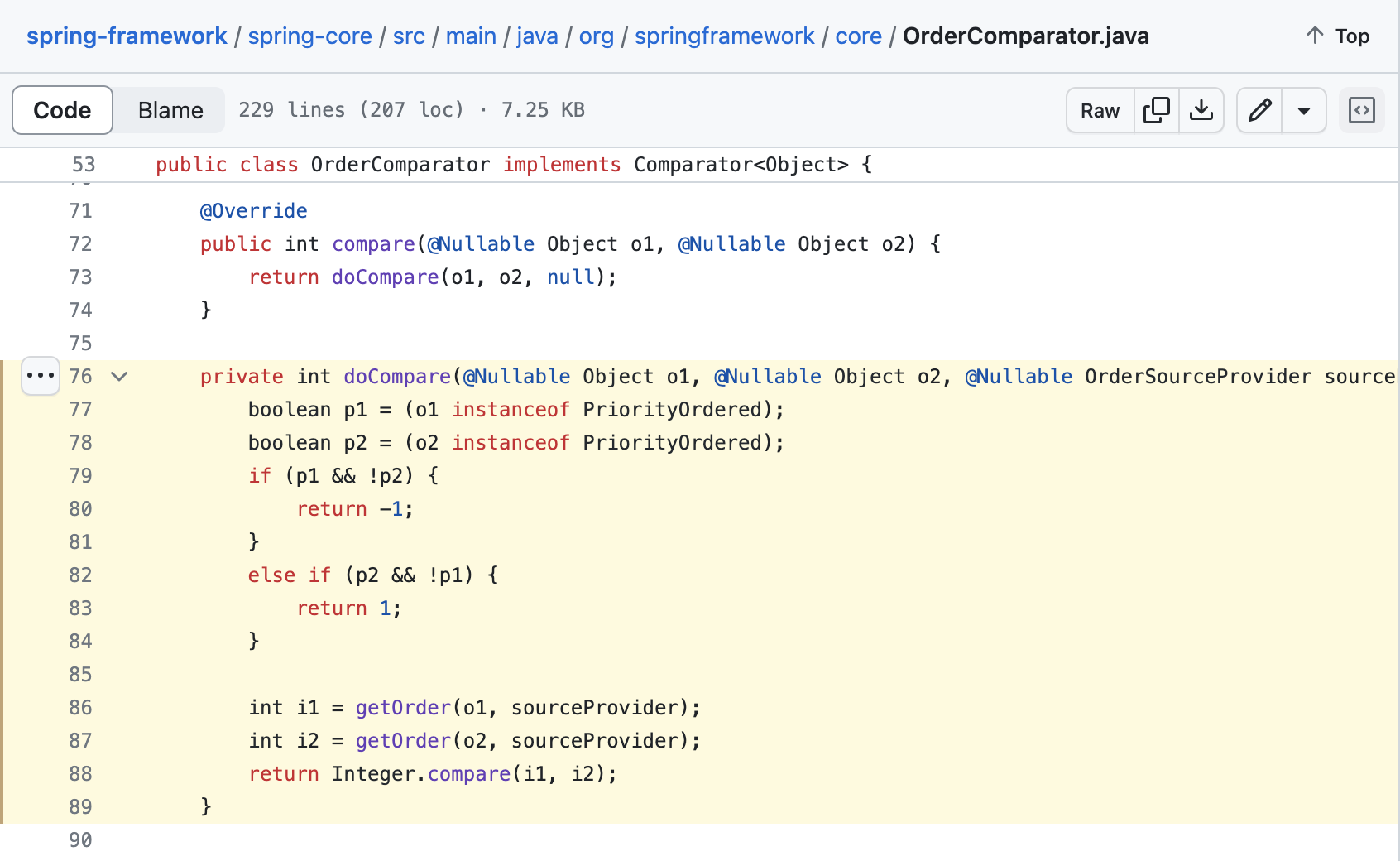``````/**
* Compares two {@code int} values numerically.
* The value returned is identical to what would be returned by:
* <pre>
*    Integer.valueOf(x).compareTo(Integer.valueOf(y))
* </pre>
*
* @param  x the first {@code int} to compare
* @param  y the second {@code int} to compare
* @return the value {@code 0} if {@code x == y};
*         a value less than {@code 0} if {@code x < y}; and
*         a value greater than {@code 0} if {@code x > y}
* @since 1.7
*/
public static int compare(int x, int y) {
return (x < y) ? -1 : ((x == y) ? 0 : 1);
}

``````

# 切量比例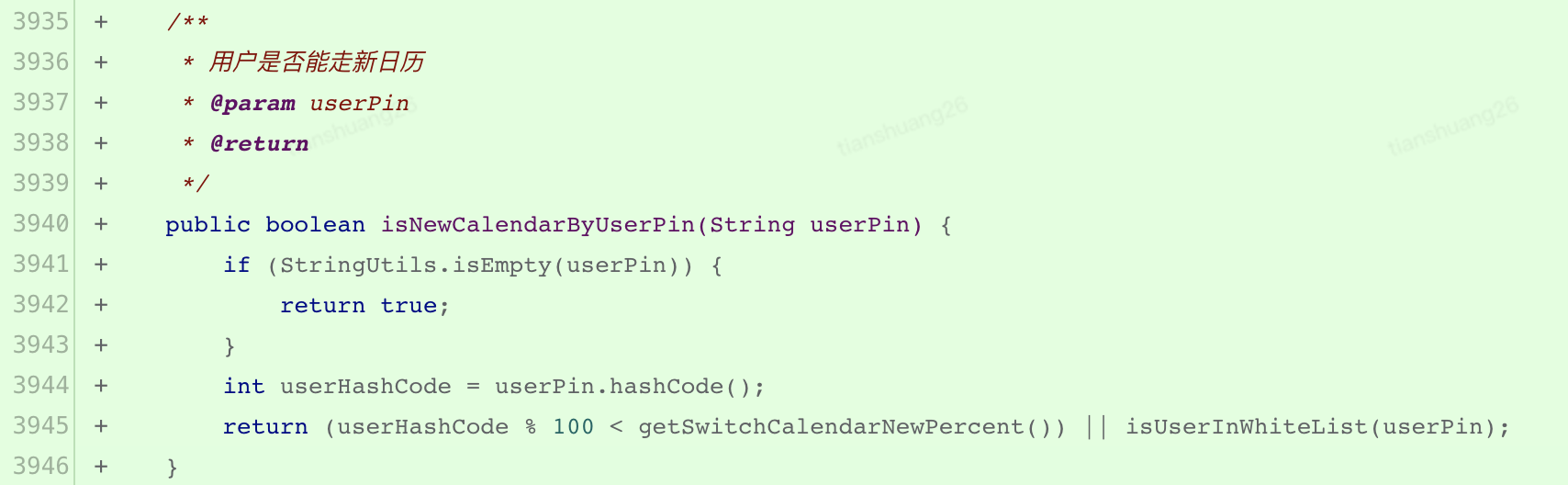``````/**
* Returns a hash code for this string. The hash code for a
* {@code String} object is computed as
* <blockquote><pre>
* s*31^(n-1) + s*31^(n-2) + ... + s[n-1]
* </pre></blockquote>
* using {@code int} arithmetic, where {@code s[i]} is the
* <i>i</i>th character of the string, {@code n} is the length of
* the string, and {@code ^} indicates exponentiation.
* (The hash value of the empty string is zero.)
*
* @return  a hash code value for this object.
*/
public int hashCode() {
int h = hash;
if (h == 0 && value.length > 0) {
char val[] = value;

for (int i = 0; i < value.length; i++) {
h = 31 * h + val[i];
}
hash = h;
}
return h;
}

``````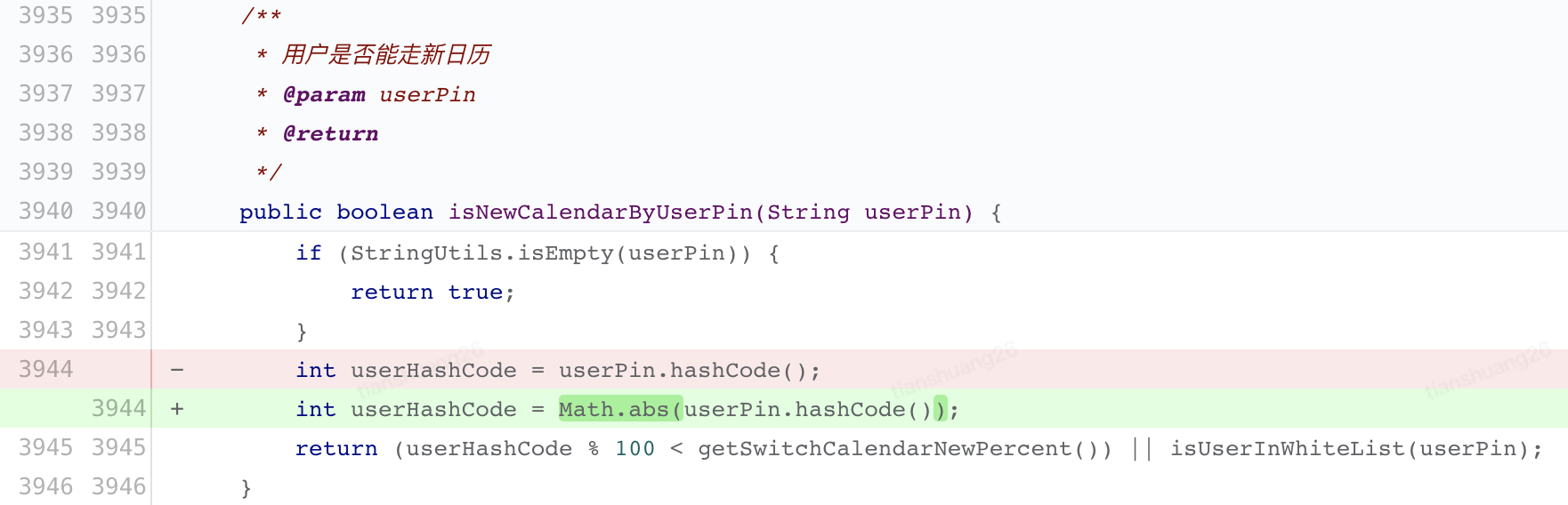``````/**
* Returns the absolute value of an {@code int} value.
* If the argument is not negative, the argument is returned.
* If the argument is negative, the negation of the argument is returned.
*
* <p>Note that if the argument is equal to the value of
* {@link Integer#MIN_VALUE}, the most negative representable
* {@code int} value, the result is that same value, which is
* negative.
*
* @param   a   the argument whose absolute value is to be determined
* @return  the absolute value of the argument.
*/
public static int abs(int a) {
return (a < 0) ? -a : a;
}

``````

``````x = Integer.MIN_VALUE:
10000000_00000000_00000000_00000000

~x:
01111111_11111111_11111111_11111111

(~x) + 1:
10000000_00000000_00000000_00000000

``````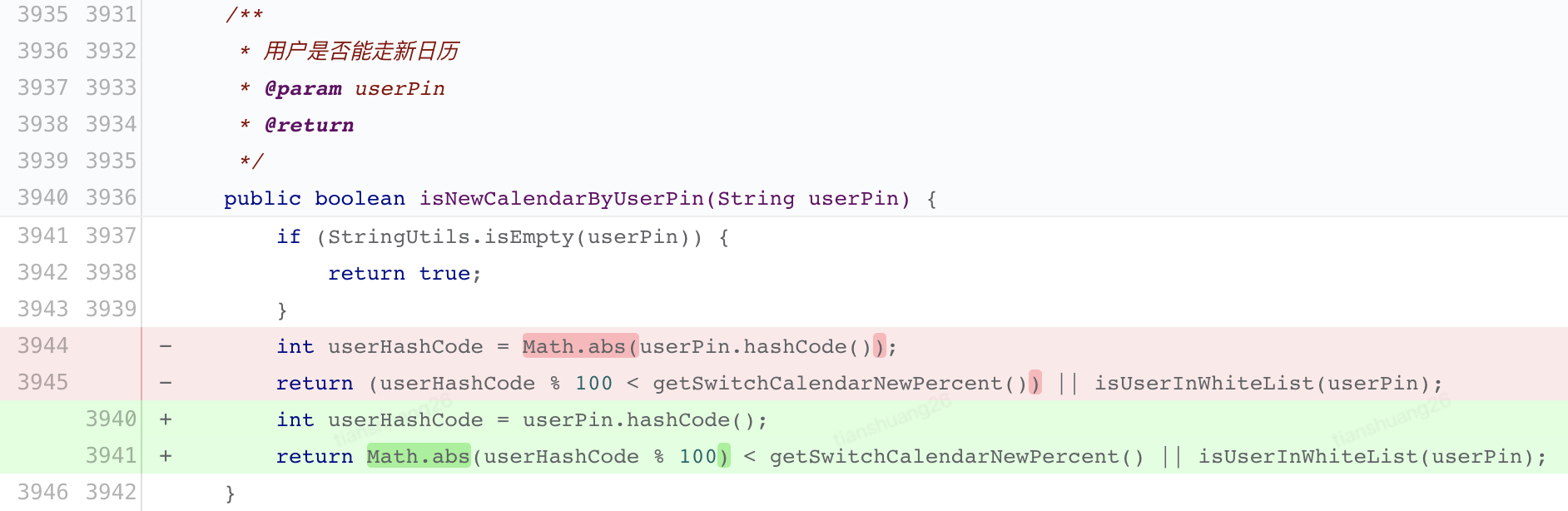# 總結

• java.lang.String#hashCode 在計算過程中可能因為整數越界導致返回值為負數
• Java 語言中的 % 是取餘而不是取模，如：(-21) % 4 = (-21) - (-21) / 4 *4 = -1
• Math.abs(int a) 當入參是 Integer.MIN_VALUE 時返回值依然是負數 Integer.MIN_VALUE

# 參考

15.15.4. Unary Minus Operator -

What's the difference between “mod” and “remainder”? - Stack Overflow

Best way to make Java's modulus behave like it should with negative numbers? - Stack Overflow

OrderComparator.java · spring-projects/spring-framework• 十二、指針和引用（二） 1、指針和數組的關係 1）思考 ​ 假設你要設計一種編程語言，你要如何實現數組呢？思考之前請先牢記：數組在記憶體中是連續的，維度由低到高（大部分操作系統下）。 2）彙編分析數組如何實現 //C++代碼 #include <iostream> int main() { int a ...
• 主要涵蓋了Spring與持久層技術的整合，以及Spring與MyBatis的整合。第一章介紹了為什麼需要將Spring與持久層技術整合，以及Spring可以與哪些持久層技術進行整合。第二章詳細介紹了Spring與MyBatis的整合思路、開發步驟和編碼實例，並解決了MyBatis開發過程中存在的問題... ...
• 背景 公司產品部收到了一些重要客戶的需求，他們希望能夠依賴獨立的資料庫存儲來支持他們的業務數據。與此同時，仍有許多中小客戶，可以繼續使用公共庫以滿足其需求。技術實現方面，此前持久層框架使用的Mybatis-plus，部分業務場景使用到了Sharding-JDBC用於分表，另外，我們的資料庫版本控制工 ...
• 近年來，車輛保險成為廣大車主必須購買的一項重要保障。然而，如何查詢車輛保險狀態及保單信息卻是許多車主面臨的難題。隨著技術的不斷發展，API的出現為我們提供了一條便捷的解決之路。本文介紹的《車輛保險查詢API——查詢車輛保險狀態及保單信息》便是一款實用的API工具。 一、API的介紹 挖數據平臺車輛保 ...
• hello，大家好！新手小白踏入 Python 的大門有點像冒險，但別擔心，我已經整理了一個超實用的入門指南，幫你規避學習過程中的十大雷區。這裡有關於 Python 的錯誤你應該註意的建議，一起來看看吧！ 1. 拼寫錯誤 小心 prin 和 print 的奇妙之旅！ # 錯誤示例 prin("Hel ...
• 通俗解釋：單例模式 > 單：唯一 > > 例：實例 > > 單例設計模式，即某個類在整個系統中只能有一個實例對象可被獲取和使用的代碼模式 > > 例如：代表JVM運行環境的Runtime類 ...
• 接上一隨筆，這次學習針對圖像數據的訪問（Numpy.array） 在OpenCV中，使用 imread() 方法可以訪問圖像，其返回值是一個數組，而根據傳入的不同圖像，將會返回不同維度的數組。 針對返回的圖像數據，即數組，我們是可以進行操作的： 1 import cv2 2 3 # MyPic.pn ...
• 在開發過程中，如果需要在本地調用openAI介面進行開發調試，一般主要是通過以下兩種方式：直連和代理轉發。歡迎私信交流。 1. 直連 1.簡單粗暴，懂的都懂 2. 代理轉發 代理轉發又有兩種類型，使用第三方代理和自建代理兩種，下麵將分別舉例說明 2.1. 第三方AI網關 1.註冊Cloudflare ...